# What is a Rotating Magnetic Field?

Posted on

When we apply a three-phase supply to a three-phase distributed winding of a rotating machine, a rotating magnetic field is produced which rotates in synchronous speed.

In this article, we will try to understand the theory behind the production of rotating magnetic field. For that, we will first imagine one stator of an electric motor where three-phase winding is physically distributed in the stator core in such a manner that winding of each phase is separated from other by 120o in space.

# What is a Rotating Magnetic Field?

Although the vector sum of three currents in a balanced three-phase system is zero at any instant, but the resultant of the magnetic fields produced by the currents is not zero rather it will have a constant non-zero value rotating in space in respect to time.

## Theory Behind the Production of Rotating Magnetic Field

The magnetic flux produced by the current in each phase can be represented by the equations given below. This is a similar representation of current is a three-phase system as the flux is cophasial with the current.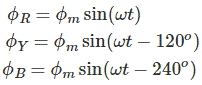Where, φR, φY and φB are the instantaneous flux of corresponding Red, Yellow and Blue phase winding, φm amplitude of the flux wave. The flux wave in the space can be represented as shown below.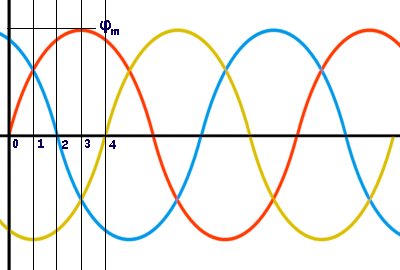Now, on the above graphical representation of flux waves, we will first consider the point 0. Here, the value of φR isThe value of φY isThe value of φB isThe resultant of these fluxes at that instant (φr) is 1.5φm which is shown in the figure below.Now, on the above graphical representation of flux waves, we will consider the point 1, where ωt = π / 6 or 30o.

Here, the value of φR is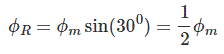The value of φY is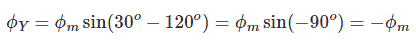The resultant of these fluxes at that instant (φr) is 1.5φm which is shown in the figure below. here it is clear thet the resultant flux vector is rotated 30o further clockwise without changing its value.Now, on the graphical representation of flux waves, we will consider the point 2, where ωt = π / 3 or 60o.

Here, the value of φR is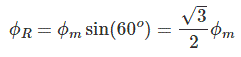The value of φY is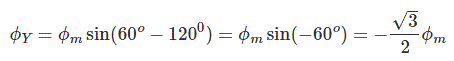The value of φB is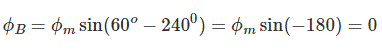The resultant of these fluxes at that instant (φr) is 1.5φm which is shown in the figure below. here it is clear thet the resultant flux vector is rotated 30° further clockwise without changing its value.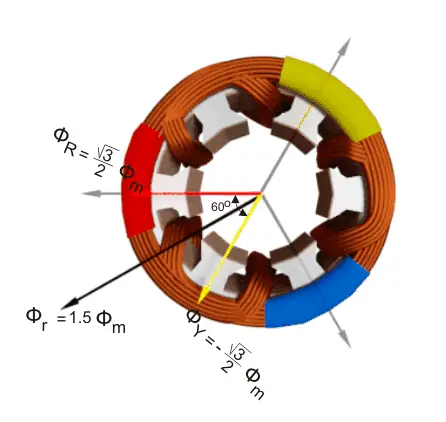Now, on the graphical representation of flux waves, we will consider the point 3, where ωt = π / 2 or 90o.

Here, the value of φR is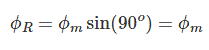The value of φY is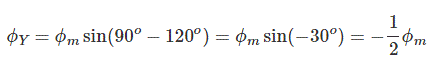The value of φB is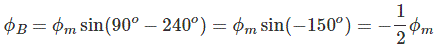The resultant of these fluxes at that instant (φr) is 1.5φm which is shown in the figure below. here it is clear thet the resultant flux vector is rotated 30o further clockwise without changing its value.In this way we can prove that the due to balanced supply applied to the three pfase stator winding a rotating or revolving magnetic fiels is established in thew space.

### Rotating Magnetic Field Conclusion

After going through the above portion of rotating magnetic field we can now establish what rotating magnetic field is. I hope you enjoy when reading this article, thank you.